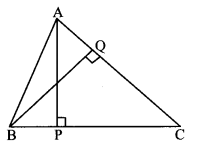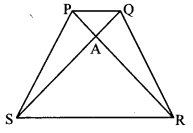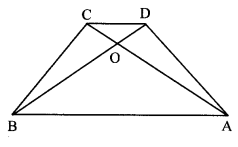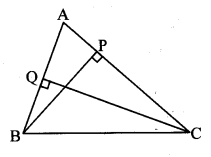# Maharashtra Board 10th Class Maths Part 2 Practice Set 1.3 Solutions Chapter 1 Similarity

Balbharti Maharashtra State Board Class 10 Maths Solutions covers the Practice Set 1.3 Geometry 10th Class Maths Part 2 Answers Solutions Chapter 1 Similarity.

## Practice Set 1.3 Geometry 10th Std Maths Part 2 Answers Chapter 1 Similarity

Practice Set 1.3 Question 1.
In the adjoining figure, ∠ABC = 75°, ∠EDC = 75°. State which two triangles are similar and by which test? Also write the similarity of these two triangles by a proper one to one correspondence.Solution:
In ∆ABC and ∆EDC,
∠ABC ≅ ∠EDC [Each angle is of measure 75°]
∠ACB ≅ ∠ECD [Common angle]
∴ ∆ABC ~ ∆EDC [AA test of similarity]
One to one correspondence is
ABC ↔ EDC

Similarity Class 10 Practice Set 1.3 Question 2.
Are the triangles in the adjoining figure similar? If yes, by which test?Solution:
In ∆PQR and ∆LMN,
$$\frac { PQ }{ LM }$$ = $$\frac { 6 }{ 3 }$$ = $$\frac { 2 }{ 1 }$$ (i)
$$\frac { QR }{ MN }$$ = $$\frac { 8 }{ 4 }$$ = $$\frac { 2 }{ 1 }$$ (ii)
$$\frac { PR }{ LN }$$ = $$\frac { 10 }{ 5 }$$ = $$\frac { 2 }{ 1 }$$ (iii)
∴ $$\frac { PQ }{ LM }$$ = $$\frac { QR }{ MN }$$ = $$\frac { PR }{ LN }$$ [From (i), (ii) and (iii)]
∴ ∆PQR – ∆LMN [SSS test of similarity]

Similarity Practice Set 1.3 Question 3.
As shown in the adjoining figure, two poles of height 8 m and 4 m are perpendicular to the ground. If the length of shadow of smaller pole due to sunlight is 6 m, then how long will be the shadow of the bigger pole at the same time?Solution:
Here, AC and PR represents the bigger and smaller poles, and BC and QR represents their shadows respectively.
Now, ∆ACB – ∆PRQ [ ∵ Vertical poles and their shadows form similar figures]
∴ $$\frac { CB }{ RQ }$$ = $$\frac { AC }{ PR }$$ [Corresponding sides of similar triangles]
∴ $$\frac { x }{ 6 }$$ = $$\frac { 8 }{ 4 }$$
∴ $$x=\frac{8 \times 6}{4}$$
∴ x = 12 m
∴ The shadow of the bigger pole will be 12 metres long at that time.

Practice Set 1.3 Geometry 10th Maharashtra Board Question 4.
In ∆ABC, AP ⊥ BC, BQ ⊥ AC, B – P – C, A – Q – C, then prove that ∆CPA – ∆CQB. If AP = 7, BQ = 8, BC = 12, then find AC.Solution:
In ∆CPA and ∆CQB,
∠CPA ≅ ∠CQB [Each angle is of measure 90°]
∠ACP ≅ ∠BCQ [Common angle]
∴ ∆CPA ~ ∆CQB [AA test of similarity]
∴$$\frac { AC }{ BC }$$ = $$\frac { AP }{ BQ }$$ [Corresponding sides of similar triangles]
∴ $$\frac { AC }{ 12 }$$ = $$\frac { 7 }{ 8 }$$
∴ AC = $$x=\frac{12 \times 7}{8}$$
∴ AC = 10.5 Units

10th Geometry Practice Set 1.3 Question 5.
Given: In trapezium PQRS, side PQ || side SR, AR = 5 AP, AS = 5 AQ, then prove that SR = 5 PQ.Solution:
side PQ || side SR [Given]
and seg SQ is their transversal.
∴ ∠QSR = ∠SQP [Altemate angles]
∴ ∠ASR = ∠AQP (i) [Q – A – S]
In ∆ASR and ∆AQP,
∠ASR = ∠AQP [From (i)]
∠SAR ≅ ∠QAP [Vertically opposite angles]
∆ASR ~ ∆AQP [AA test of similarity]
∴ $$\frac { AS }{ AQ }$$ = $$\frac { SR }{ PQ }$$ (ii) [Corresponding sides of similar triangles]
But, AS = 5 AQ [Given]
∴ $$\frac { AS }{ AQ }$$ = $$\frac { 5 }{ 1 }$$ (iii)
∴ $$\frac { SR }{ PQ }$$ = $$\frac { 5 }{ 1 }$$ [From (ii) and (iii)]
∴ SR = 5 PQ

Practice Set 1.3 Geometry 10th Question 6.
Id trapezium ABCD (adjoining figure), side AB || side DC, diagonals AC and BD intersect in point O. If AB = 20, DC = 6, OB = 15, then find OD.Solution:
side AB || side DC [Given]
and seg BD is their transversal.
∴ ∠DBA ≅ ∠BDC [Alternate angles]
∴ ∠OBA ≅ ∠ODC (i) [D – O – B]
In ∆OBA and ∆ODC
∠OBA ≅ ∠ODC [From (i)]
∠BOA ≅ ∠DOC [Vertically opposite angles]
∴ ∆OBA ~ ∆ODC [AA test of similarity]
∴ $$\frac { OB }{ OD }$$ = $$\frac { AB }{ DC }$$ [Corresponding sides of similar triangles]
∴ $$\frac { 15 }{ OD }$$ = $$\frac { 20 }{ 6 }$$
∴ OD = $$x=\frac{15 \times 6}{20}$$
∴ OD = 4.5 units

Class 10 Geometry Practice Set 1.3 Question 7.
꠸ ABCD is a parallelogram. Point E is on side BC. Line DE intersects ray AB in point T. Prove that DE × BE = CE × TE.Solution:
Proof:
꠸ ABCD is a parallelogram. [Given]
∴ side AB || side CD [Opposite sides of a parallelogram]
∴ side AT || side CD [A – B – T]
and seg DT is their transversal.
∴ ∠ATD ≅ ∠CDT [Alternate angles]
∴ ∠BTE ≅ ∠CDE (i) [A – B – T, T – E – D]
In ∆BTE and ∆CDE,
∠BTE ≅ ∠CDE [From (i)]
∠BET ≅ ∠CED [Vertically opposite angles]
∴ ∆BTE ~ ∆CDE. [AA test of similarity]
∴ $$\frac { TE }{ DE }$$ = $$\frac { BE }{ CE }$$ [Corresponding sides of similar triangles]
∴ DE × BE = CE × TE

Geometry Practice Set 1.3 Question 8.
In the adjoining figure, seg AC and seg BD intersect each other in point P and $$\frac { AP }{ CP }$$ = $$\frac { BP }{ DP }$$ Prove that, ∆ABP ~ ∆CDPSolution:
Proof:
In ∆ABP and ∆CDP,
$$\frac { AP }{ CP }$$ = $$\frac { BP }{ DP }$$ [Given]
∠APB ≅ ∠CPD [Vertically opposite angles]
∴ ∆ABP ~ ∆CDP [SAS test of similarity]

Math 2 Practice Set 1.3 Question 9.
In the adjoining figure, in ∆ABC, point D is on side BC such that, ∠BAC = ∠ADC. Prove that, CA2 = CB × CD,Solution:
Proof:
∠BCA ≅ ∠ACD [Common angle]
∴ ∆BAC ~ ∆ADC [AA test of similarity]
∴ $$\frac { CA }{ CD }$$ = $$\frac { CB }{ CA }$$ [Corresponding sides of similar triangles]
∴ CA × CA = CB × CD
∴ CA2 = CB × CD

Question 1.
In the adjoining figure, BP ⊥ AC, CQ ⊥ AB, A – P – C, A – Q – B, then prove that ∆APB and ∆AQC are similar. (Textbook pg. no. 20)Solution:2. SAS test for similarity of triangles:
For a given correspondence, if two pairs of corresponding sides are in the same proportion and the angle between them is congruent, then the two triangles are similar.In the given figure, if $$\frac { AB }{ PQ }$$ = $$\frac { BC }{ QR }$$, and ∠B ≅∠Q, then ∆ABC ~ ∆PQR

3. SSS test for similarity of triangles:
For a given correspondence, if three sides of one triangle are in proportion with the corresponding three sides of the another triangle, then the two triangles are similar.In the given figure, if $$\frac { AB }{ PQ }$$ = $$\frac { BC }{ QR }$$ = $$\frac { AC }{ PR }$$, then ∆ABC ~ ∆PQR

Properties of similar triangles:

1. Reflexivity: ∆ABC ~ ∆ABC
2. Symmetry : If ∆ABC ~ ∆DEF, then ∆DEF ~ ∆ABC.
3. Transitivity: If ∆ABC ~ ∆DEF and ∆DEF ~ ∆GHI, then ∆ABC ~ ∆GHI.
Scroll to Top